# 1952~2018年陕西省夏季温度、降水、风速演变的城郊对比Comparison of the Evolution of Temperature, Precipitation and Wind Speed between City and Suburb in Summer of Shaanxi Province from 1952 to 2018

DOI: 10.12677/CCRL.2020.92006, PDF, HTML, XML, 下载: 254  浏览: 617

Abstract: In the process of urban development, the underlying surface of urban areas will have a significant impact on climate change. In this paper, the monthly climatological data of Yulin and Hanzhong stations in Shaanxi Province from 1952 to 2018 were used to analyze the changing trends of air temperature, precipitation and wind speed in urban and suburban areas of Shaanxi Province through Morlet wavelet analysis and sliding t-test. In addition, according to the time series of precipitation in Hanzhong, Morlet wavelet analysis is carried out for the obtained short time series and comparison is made. The results show that compared with Hanzhong, Yulin has lower temperature, less precipitation and higher wind speed. The temperature and wind speed of the two places showed an upward trend, but the precipitation in Hanzhong showed a downward trend, while the precipitation in Yulin showed an upward trend. The change rate of meteorological elements in Hanzhong is more obvious than that in Yulin. The time scales of temperature, precipitation and wind speed are not consistent. From the perspective of time scale, considering the boundary effects, the summer precipitation in Hanzhong has a 5-year cycle, while the summer precipitation in Yulin has no obvious time scale characteristics. The summer temperature in Hanzhong has no obvious time-scale characteristics, while the summer temperature in Yulin has a 2-year cycle, a 5-year cycle and an 8-year cycle. The summer wind speed in Hanzhong and Yulin has no obvious time scale characteristics. In addition, the decomposition of sequences has an impact on the results of wavelet analysis. The period before partial years is not obvious, while the time scale features are obvious after partial years.

1. 引言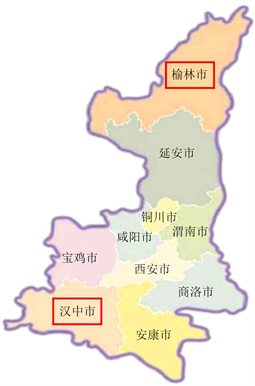Figure 1. Map of Shaanxi Province with Yulin and Hanzhong in red boxes (The image is from http://image.so.com/)

2. 数据和方法

2.1. 数据

2.2. 小波分析

2.2.1. 母小波

Morlet小波为复数小波，包含了实部虚部。表达式如下

$\Psi \left(u\right)=C{\text{e}}^{-i{\omega }_{0}u}\left({\text{e}}^{-\frac{{u}^{2}}{2}}-\sqrt{2}{\text{e}}^{-\frac{{\omega }_{0}^{2}}{4}}{\text{e}}^{-{u}^{2}}\right)$

${\int }_{-\infty }^{+\infty }{|\Psi \left(u\right)|}^{2}du=1$

2.2.2. 子波

${\int }_{-\infty }^{+\infty }\Psi \left(T\right)\text{d}t=0,\Psi \left(T\right)\in {L}^{2}\left(R\right)$

${\Psi }_{a,b}\left(t\right)={|a|}^{-\frac{1}{2}}\Psi \left(\frac{t-b}{a}\right),a,b\in R,a\ne 0$

2.2.3. 小波变换

$\begin{array}{c}{W}_{f}\left(a,b\right)\equiv {\int }_{-\infty }^{+\infty }{f}_{\left(t\right)}{\Psi }_{a,b\left(t\right)}^{\ast }\text{d}t={\int }_{-\infty }^{+\infty }\left[\frac{1}{2\text{π}}{\int }_{-\infty }^{+\infty }{f}_{\left(\omega \right)}{\stackrel{^}{e}}^{i\omega t}\text{d}\omega \right]{|a|}^{-\frac{1}{2}}{\Psi }^{\ast }\left(\frac{t-b}{a}\right)\text{d}t\\ =\frac{1}{2\text{π}}{|a|}^{-\frac{1}{2}}{\int }_{-\infty }^{+\infty }{\stackrel{^}{f}}_{\left(\omega \right)}{\stackrel{^}{\Psi }}_{\left(a\omega \right)}^{\ast }{\text{e}}^{i\omega b}\text{d}\omega \\ =\frac{1}{2\text{π}}{\int }_{-\infty }^{+\infty }{\stackrel{^}{f}}_{\left(\omega \right)}{\stackrel{^}{\Psi }}_{\left(a\omega \right)}^{\ast }\text{d}\omega \end{array}$

2.2.4. 小波功率谱边界效应及边界影响e-折曲线

3. 降水序列趋势分析及小波分析

3.1. 降水序列趋势分析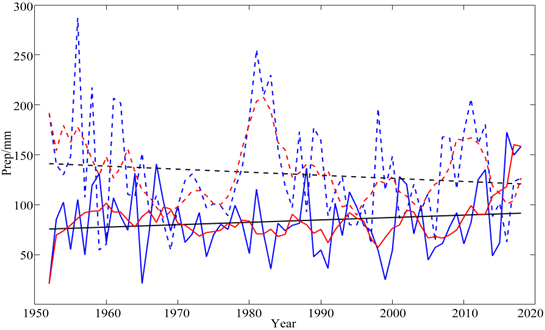Figure 2. Time series of summer precipitation from 1952 to 2018. The dotted line represents Hanzhong and the solid line represents Yulin. Blue represents the original sequence, black represents unitary linear fitting sequence, and red represents sliding average sequence

3.2. 降水序列小波分析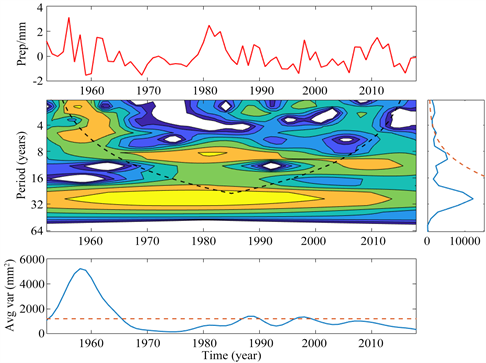Figure 3. Morlet wavelet analysis of Hanzhong summer precipitation sequence from 1952 to 2018. Above: standardized time series of summer precipitation anomaly (solid red line); Middle left: wavelet power spectrum, black dotted line represents e-folded curve of boundary influence; Center right: time average spectrum (solid blue line), dotted red line is 0.05 confidence line; Bottom: 2~8 years average spectrum (solid blue line), dotted red line is 0.05 confidence line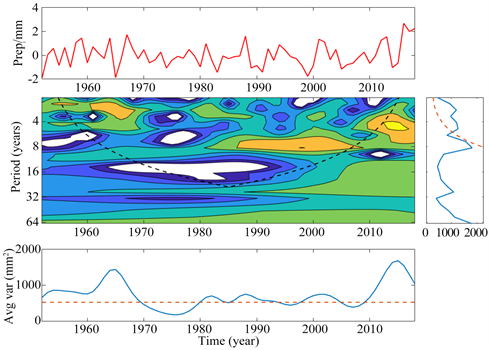Figure 4. Same as Figure 3, but Morlet wavelet analysis diagram of Yulin summer precipitation sequence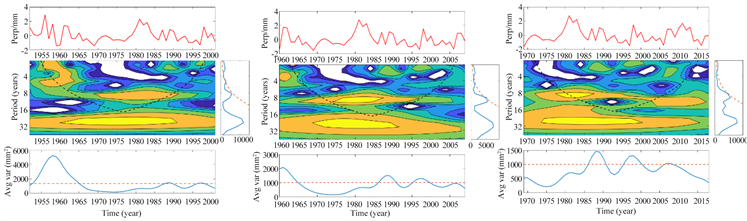Figure 5. Wavelet analysis diagram of the sequence (length of 50 years) of Hanzhong summer precipitation after decomposition. Left: 1952~2001; middle: 1960~2009; right: 1969~2018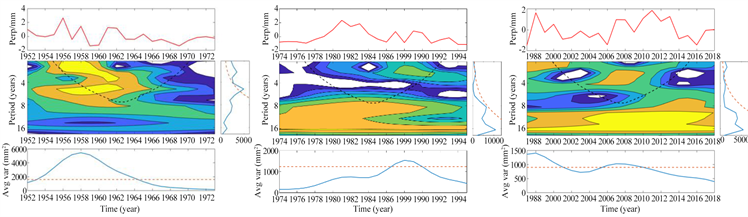Figure 6. Wavelet analysis diagram of the sequence (length of 50 years) of Hanzhong summer precipitation after decomposition. Left: 195~1973, center: 1974~1995, right: 1997~2018

4. 气温序列趋势分析及小波分析

4.1. 气温序列趋势分析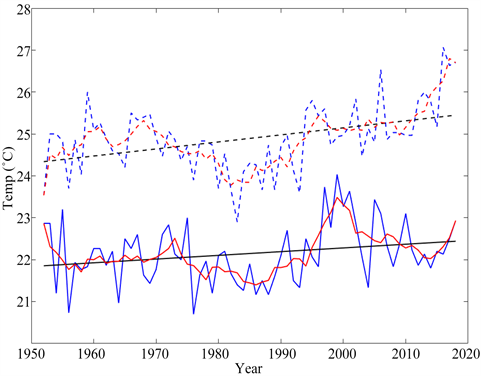Figure 7. Time series of summer temperature from 1952 to 2018. The dotted line represents Hanzhong and the solid line represents Yulin. Blue represents the original sequence, black represents unitary linear fitting sequence, and red represents sliding average sequence

4.2. 气温序列小波分析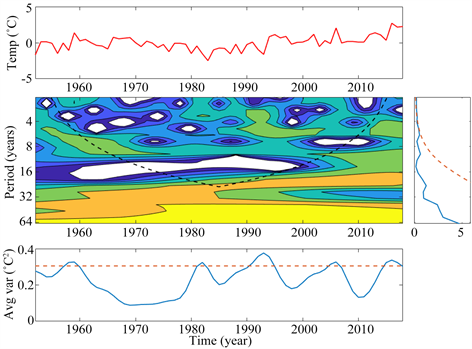Figure 8. Morlet wavelet analysis of summer temperature sequence in Hanzhong from 1952 to 2018. Above: standardized time series of summer temperature anomaly (solid red line); Middle left: wavelet power spectrum, black dotted line represents e-folded curve of boundary influence; Center right: time average spectrum (solid blue line), dotted red line is 0.05 confidence line; Bottom: 2~8 years average spectrum (solid blue line), dotted red line is 0.05 confidence line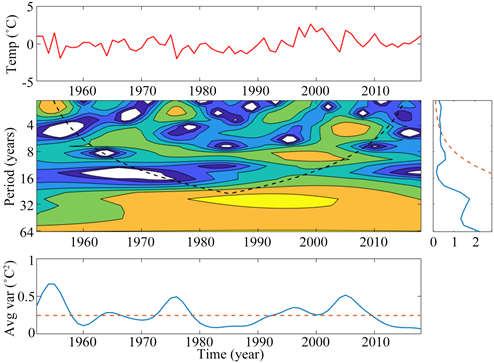Figure 9. Same as Figure 8, but Morlet wavelet analysis diagram of Yulin summer temperature sequence

5. 风速序列趋势分析及小波分析

5.1. 风速序列趋势分析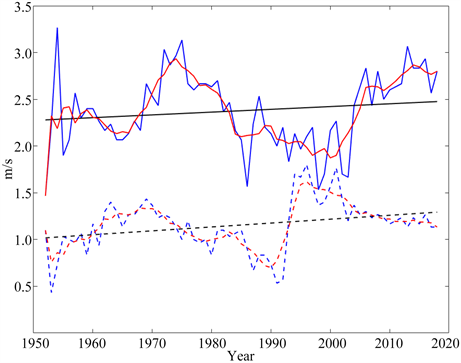Figure 10. Summer wind speed time series 1952~2018. The dotted line represents Hanzhong and the solid line represents Yulin. Blue represents the original sequence, black represents unitary linear fitting sequence, and red represents sliding average sequence

5.2. 风速序列小波分析Figure 11. Morlet wavelet analysis of Hanzhong summer wind speed sequence from 1952 to 2018. Above: standardized time series of summer wind speed anomaly (solid red line); Middle left: wavelet power spectrum, black dotted line represents e-folded curve of boundary influence; Center right: time average spectrum (solid blue line), dotted red line is 0.05 confidence line; Bottom: 2~8 years average spectrum (solid blue line), dotted red line is 0.05 confidence line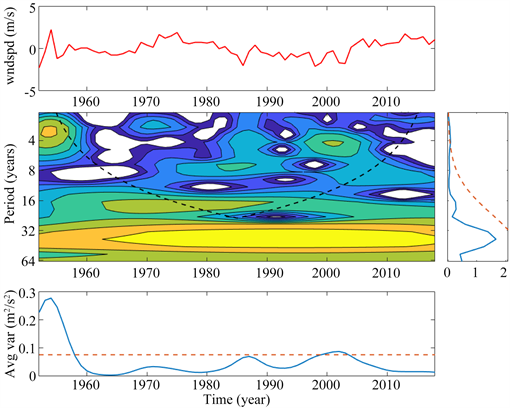Figure 12. Same as Figure 11, but Morlet wavelet analysis diagram of Yulin summer wind speed sequence

6. 结论及展望

1) 气象要素平均状态城郊对比来看，汉中降水明显多于榆林，汉中气温高于榆林，汉中风速明显小于榆林；

2) 气象要素变化趋势城郊对比来看，汉中降水有缓慢减小的趋势，而榆林降水呈现缓慢增大的趋势。两地气温均有缓慢上升的趋势，两地风速均有缓慢增大的趋势；

3) 时间尺度城郊对比来看，考虑边界效应，汉中夏季降水为准5年周期，榆林夏季降水没有明显的时间尺度特征。汉中夏季气温没有明显的周期特征，榆林夏季气温为准2年周期、准5年周期和准8年周期。汉中夏季风速以及榆林夏季风速均没有明显的周期特征；

4) 汉中降水序列拆分后进行小波分析的结果表明，部分年份拆分前周期不明显，拆分后出现明显的时间尺度特征。

1) 郊区和城区各仅用一个气象站代替，气象站个数较少，结果可信度存疑；

2) 汉中相对榆林纬度偏南4˚左右，在气温序列分析中，未能剔除纬度的影响。

  杨萍, 刘伟东, 侯威. 北京地区城郊极端温度事件的变化趋势及差异分析[J]. 气候与环境研究, 2013, 18(1): 80-86.  宋艳玲, 董文杰, 张尚印, 张德宽, 王守荣, 胡宝昆. 北京市城、郊气候要素对比研究[J]. 干旱气象, 2003, 21(3): 63-68.  李淼, 夏军, 陈社明, 孟德娟. 北京地区近300年降水变化的小波分析[J]. 自然资源学报, 2011, 26(6): 1001-1011.  程胜龙, 王乃昂. 近六、七十年来兰州城市发展对城市气候环境的影响[J]. 城市环境与城市生态, 2004, 17(4): 28-31.  周丽英, 杨凯. 上海降水百年变化趋势及其城郊的差异[J]. 地理学报, 2001, 56(4): 467-472.  李杨, 张小曳, 车慧正, 陈广善, 屈文军. 城市化效应对西安地区气候的影响[C]//第八届全国气溶胶会议暨第二届海峡两岸气溶胶科技研讨会, 2005.  陈伟, 汤静, 卜思阳, 王晶晶. 基于Mann-Kendall法和小波分析的沈阳站1951-2010年降水变化特征研究[J]. 农业灾害研究, 2015, 5(11): 37-38.  娄德君, 孙卫国, 李治民. 齐齐哈尔市气温变化的小波分析[J]. 气象科技, 2004, 32(6): 475-478.  刘鸿飞, 景元书, 马林. 铜陵市近50年气温变化的小波分析[J]. 安徽农业科学, 2010, 38(31): 17613-17616+17620.  魏凤英. 现代气候统计诊断预测技术[M]. 北京: 气象出版社, 1999: 194-201.  吴国曾. 实时信号小波分析中边界处理方法及应用[D]: [硕士学位论文]. 河南: 郑州大学电气工程系, 2010.